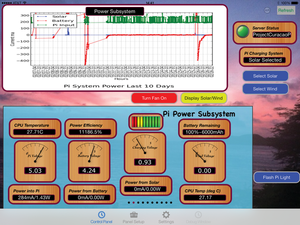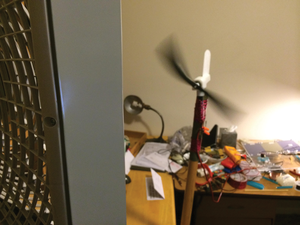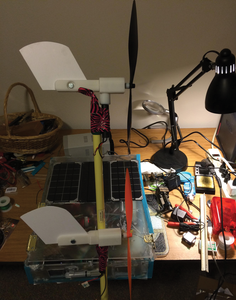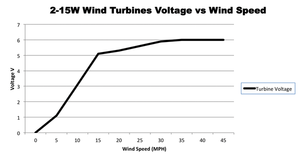# Sizing a wind turbine to power your Raspberry Pi

#### The Design

These inexpensive wind turbines have no specifications to speak of. The 15W turbines are rated at 15W (12V at 1.25A), but the documentation tells nothing about how fast the wind needs to be or what voltages the turbine can generate.

The 50W Turbine (25V at 2.0A) documentation says nothing about the dynamic characteristics of the turbine – no curves or suggestion of what wind speeds could make the 50W numbers work.

Therefore, I needed to generate data measurements and plot curves to make some rational decisions about how to integrate the turbines into the design.

I performed two measurements on both devices. An Open Load (or Open Loop) characterization, with the turbine spinning freely, provided an indication about what the wind speed needs to be to generate enough voltage (actually the minimum possible speed, because there is no load).

A Loaded characterization offered some insight into the actual power needed for the project. I used the actual device to provide the load for this test.

I calculated roughly a 25-ohm effective load from the device at minimum resistance (maximum current) by looking at the current from a power supply. I monitor and control the power system with the RasPiConnect app  (Figure 6). Note that the power efficiency number in Figure 6 is wrong because the unit is plugged into the mains.Figure 6: You can manage the Raspberry Pi through the RasPiConnect control panel.

For the Open Load characterization, I measured the unregulated wind turbine voltage, the regulated wind turbine voltage, the Raspberry Pi solar panel voltage, and the corresponding currents using INA219 high-side current and voltage i2c sensors.

The 15W wind turbine is a US\$ 90 single-blade, 15W, DIY small wind turbine kit system . You also can find this device on Amazon as a Micro Wind Turbine Generator Science Project Kit for US\$ 40, but without the connecting wires.

The 50W wind turbine is a Cyber 50 Micro wind turbine generator purchased from Amazon.com for US\$ 70.

I'm not specifically recommending either of these turbines, but they are the first inexpensive ones that I ran across.

The first thing I needed to do was characterize the open loop voltage curve so that I knew what voltages I was getting out of the turbine.

So, I mounted the 15W turbine on a garden spade (literally – Figure 7) and stuck it outside the car through the window and drove at fixed rates of speed and measured the voltage with a multimeter (Figure 8). I got some strange looks from the neighbors (and a policeman) and had a hard time maintaining the speed because of a blizzard and ice on the road.Figure 7: The 15W wind turbine mounted on a spade.Figure 8: Taking the 15W wind turbine for a drive in a snowstorm.

I gathered the data up to 50mph, put it in an Excel spreadsheet, and did a third order polynomial curve fit to get an equation to use in the software to predict the wind speed given an open loop voltage (Figure 9).Figure 9: Voltage versus wind speed for the 15W wind turbine open loop.

The equation I generated was:

where y = wind turbine output voltage and x = wind speed in miles per hour (mph).

The coefficients are:

c3 = -0.000224491

c2 = 0.012549184

c1 = 0.255523699

b = 0.247342657

The maximum curve fit errors are +0.4V/-0.6V, +6.89%/-4.74%, which was good enough for my purposes. The data certainly wasn't any better than +/-10 percent given the snow and general driving. Note that this curve is useful for control functions inside the Raspberry Pi, and for calculating the wind speed, but it is only valid for open loop.

Based on a small amount of data (during a wind storm one night, I put the wind turbine outside), I determined that I needed an additional turbine to generate power in low wind conditions (e.g., 10-14mph). I came to this conclusion by looking at the amount of voltage and current generated by the wind storm when the wind was blowing around 15mph. From the RasPiConnect control panel (Figure 10), you can see that very little current was generated by one 15W turbine. The blue dots represent the regulated voltage going into the battery charging circuitry. The voltage needs to be above 5V to generate any significant current to the battery. Even though the storm generated winds of more than 15mph, the charger rarely turned on. The higher voltage dots to the right of the graph were generated by taking the entire box and the turbine in a car to verify this conclusion.Figure 10: Very little current is generated by one 15W turbine.

I subsequently purchased an additional 15W turbine, and the open loop characteristics look the same with both in parallel. The first measurements I made with both 15W turbines indicated that the configuration might meet my power requirements. However, I later determined this design was marginal by examining the loaded voltage curves for the dual turbine.

I needed a minimum 9V to run into the 6V regulator (which then is fed to the battery charging circuit). Figure 11 shows the two 15W wind turbine test jig, and the 50W turbine is shown in Figure 12.Figure 11: The two 15W wind turbine test jig.

I gathered the data up to 50mph, put it in an Excel spreadsheet, and did a third order polynomial curve fit to get an equation to predict the wind speed given an open loop voltage (Figure 13).Figure 13: Voltage versus wind speed for the 50W wind turbine open loop.

The equation generated was:

where y = wind turbine output voltage, and x = wind speed in miles per hour.

The coefficients are:

c3 =-0.000385082

c2 = 0.022102564

c1 = 0.675897436

b = 0.172727273

The maximum curve-fit errors are +0.9V/-1.07V, +6.75%/-7.77%.

My initial conclusion was that either the two 15W turbines or the 50W turbine would meet my needs. I came to this conclusion by looking at the open loop graphs and reading the voltage at 15mph. Fifteen miles per hour is the target wind speed for Curacao based on weather charts. The 15W open loop chart gives about 5.8V at 15mph; the 50W open loop chart gives about 13V. At 5.8V, the regulator should turn on, but at a low efficiency. This was verified by using a variable power supply. However, the loaded tests show that the dual 15W turbine does not generate enough current to turn on the regulator (Figure 14).Figure 14: Loaded voltage versus wind speed for the double 15W turbine configuration.

Next, I did the loaded test of both configurations in the Project Curacao box (with the Pi reporting data) in the actual circuit.

Express-Checkout as PDF

Pages: 8

Price \$2.95
(incl. VAT)